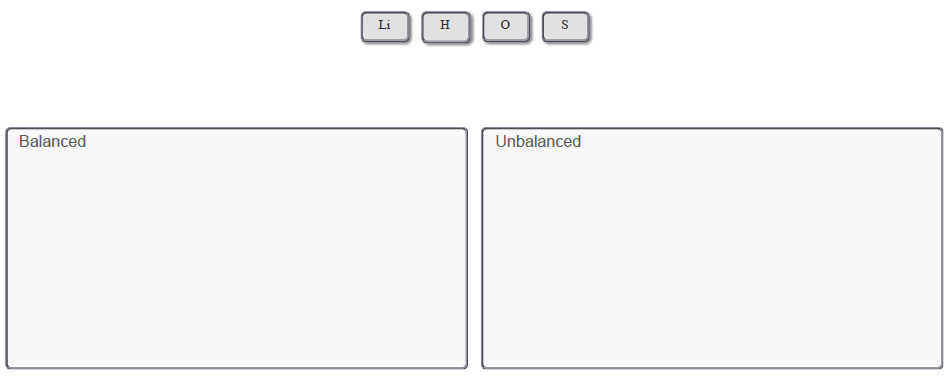# Problem: Determine which elements are balanced and which are unbalanced in the following partially balanced chemical equation.2LiOH + H2SO4 ⟶ Li2SO4 + H2ODrag the appropriate items to their respective bins.Coefficients in chemical equationsCoefficients in chemical equations are the numbers placed before molecules or ions to balance the amount of atoms of each element on both sides of the equation. For example, a coefficient of 2 in front of NaCl indicates that there are two Na atoms and two Cl atoms in the balanced equation. When a molecule contains a subscript, the subscript must also be considered. For example, a coefficient of 4 in front of CH4 indicates that there are four carbon atoms and 4 × 4 = 16 hydrogen atoms. The subscript is multiplied by the coefficient to get the total number. When the subscript is contained outside parentheses, the 2 in 3Ca(OH)2 for example, it is applied to each atom contained in the parentheses. So, the total number of oxygen atoms in 3Ca(OH)2 is six and the total number of hydrogen atoms in 3Ca(OH)2 is six.

###### FREE Expert SolutionView Complete Written Solution
###### Problem Details

Determine which elements are balanced and which are unbalanced in the following partially balanced chemical equation.

2LiOH + H2SO4 ⟶ Li2SO4 + H2O

Drag the appropriate items to their respective bins.Coefficients in chemical equations

Coefficients in chemical equations are the numbers placed before molecules or ions to balance the amount of atoms of each element on both sides of the equation. For example, a coefficient of 2 in front of NaCl indicates that there are two Na atoms and two Cl atoms in the balanced equation. When a molecule contains a subscript, the subscript must also be considered. For example, a coefficient of 4 in front of CH4 indicates that there are four carbon atoms and 4 × 4 = 16 hydrogen atoms. The subscript is multiplied by the coefficient to get the total number. When the subscript is contained outside parentheses, the 2 in 3Ca(OH)2 for example, it is applied to each atom contained in the parentheses. So, the total number of oxygen atoms in 3Ca(OH)2 is six and the total number of hydrogen atoms in 3Ca(OH)2 is six.